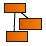gl_normalize

• Where to find it:

•NodeGraph / Right Mouse Click / Add Nodes / Math / Extension Pack / N /

 Description

normalize returns a vector with the same direction as its parameter, v, but with length 1.

 OpenGl Reference

https://www.khronos.org/registry/OpenGL-Refpages/gl4/html/normalize.xhtml

 Node Ports

• Vector

Specify the Vector to Normalize

 Node Outputs

• Output RGB

computation for Input Channels Red, Green, Blue with an Alpha Channel of 1.0

Output = vec4( normalize(X.rgb),1.0)

• Output RGBA

computation for Input Channels Red, Green, Blue and Alpha

Output = normalize(X)

• R

Computation for Input Red channel only, saved into R, G and B channel with an Alpha of 1.0

r = normalize(X.r)

Output = vec4(r,r,r,1.0)

• G

Computation for Input Green channel only, saved into R, G and B channel with an Alpha of 1.0

g = normalize(X.g)

Output = vec4(g,g,g,1.0)

• B

Computation for Input Blue channel only, saved into R, G and B channel with an Alpha of 1.0

b = normalize(X.b)

Output = vec4(b,b,b,1.0)

• A

Computation for Input Alpha channel only, saved into R, G and B channel with an Alpha of 1.0

a = normalize(X.a)

Output = vec4(a,a,a,1.0)